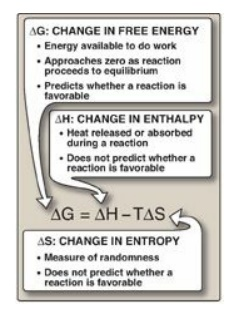# Free Energy

| Home | | Biochemistry |

## Chapter: Biochemistry : Bioenergetics and Oxidative Phosphorylation

The direction and extent to which a chemical reaction proceeds is determined by the degree to which two factors change during the reaction.

FREE ENERGY

The direction and extent to which a chemical reaction proceeds is determined by the degree to which two factors change during the reaction. These are enthalpy (∆H, a measure of the change in heat content of the reactants and products) and entropy (∆S, a measure of the change in randomness or disorder of reactants and products; Figure 6.1). Neither of these thermodynamic quantities by itself is sufficient to determine whether a chemical reaction will proceed spontaneously in the direction it is written. However, when combined mathematically (see Figure 6.1), enthalpy and entropy can be used to define a third quantity, free energy (G), which predicts the direction in which a reaction will spontaneously proceed.Figure 6.1 Relationship between changes in free energy (G), enthalpy (H), and entropy (S). T is the absolute temperature in Kelvin (K): K = oC + 273.